The differential equation for the Zaev effect in capacitances or inductivities
Part I: the differential equation of the effect

Acc. to a previous message on this server Nicolay Zaev discovered experimentally a probably electro-caloric overunity effect in capacities and inductivities. This work was based on work of Golitcin from last century who seems to have investigated dielectrics whose dielectric material constant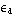r depended strongly from temperature. (Sorry, I can not cite the original literature correctly lack of any knowledge of the Russian language). However, it was criticized that the theory of Zaev seemed not to be useful to describe the effect, because it did not contained any thermodynamic material parameter. Sergej Godin took up the thermodynamic ideas and built up a good and simple test-setup useful to prove that appropriately chosen ratched like asymmetric voltage charge-discharge potential forms produce the effect in capacitances. In the answer of his success message I proposed a possible electro-caloric cycle whose differential equation will be developed here. In order to understand basic principles we develop here a simplified differential equation of the problem which reproduces correctly the qualitative features of the experiment. In order to do the first step we assume for sake of simplicity that the capacitive dielectrics has no piezomechanic movement, no hysteresis and no energy dissipation - all things which exist in reality but complicate here the problem too much because we want to see here only some aspects.

The free energy differential dF of the dielectrics is therefore (V=volume, E=electric field, P=polarisation, S=entropy, and T=temperature)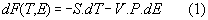with S = -dF/dT and V. P = -dF/dE . Because the problem is dependent from E and T we can write the total differential of entropy as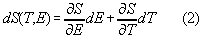Acc. to the Maxwell relations of this potential it holds CE = T .(dS /dT)E and (dS /dE)T = V . (dP/dT)E . These relations can be inserted into the equation above and we get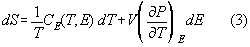If dS=0, i.e. no heat exchange, we get the equation of the isentrope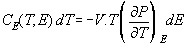Under our general conditions dS is not zero generally. In order to get the differential equation we parametrize the total entropy differential with respect to the time t using the Maxwell-relations we can rewrite equation (3)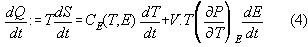where we identified the heat flux dQ/dt by dQ/dt:= T .dS/dt. If we remember the thermal analog on Ohm's law for the heat current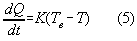(with Te :=temperature of the environment and K:=heat conduction coefficient of the dielectrics to environment) and insert (5) in eq.(4) then we get a general differential equation of first order for this problem.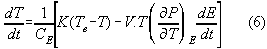Now, we have to specify the constitutive equation of state for the dielectrics under consideration.
We consider only small signal amplitude of E . Therefore, we can restrict us to first order terms and describe the dielectric susceptibility to be only linear dependent from T. (It is clear that in reality a scaling law |T-Tc| -with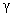>0 would be appropriate, however this makes only sense if concrete experimental values exist for the problem under consideration.)

The free energy function of the material can be written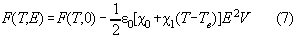Therefrom, using a Maxwell equation, the entropy of the system followsand similar, using a standard formula, specific heat of the substance follows as well.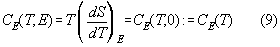Therefrom, using the Maxwell relation dS/dE = V.dP/dT, and assuming that CE(T)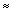CE(TE):=CE we get the differential equation specified for the linear material.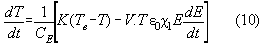Now, we specify an analytical test signal E(t) applied to the dielectrics as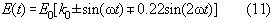This signal form is a relatively simple analytical offset including sawtooth sinus whose fast slopes interchanges with the slow ones if the upper or lower signs are used respectively. It is inserted into eq.10 and, using the definitions x:=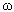t, :=K/(.CE), T':=T-Te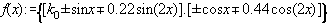and q:=V.0.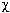1.E02 /CE , we get the linear inhomogeneous differential equation describing the problem.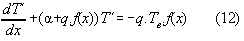This equation we solve numerically.

(to be continued)